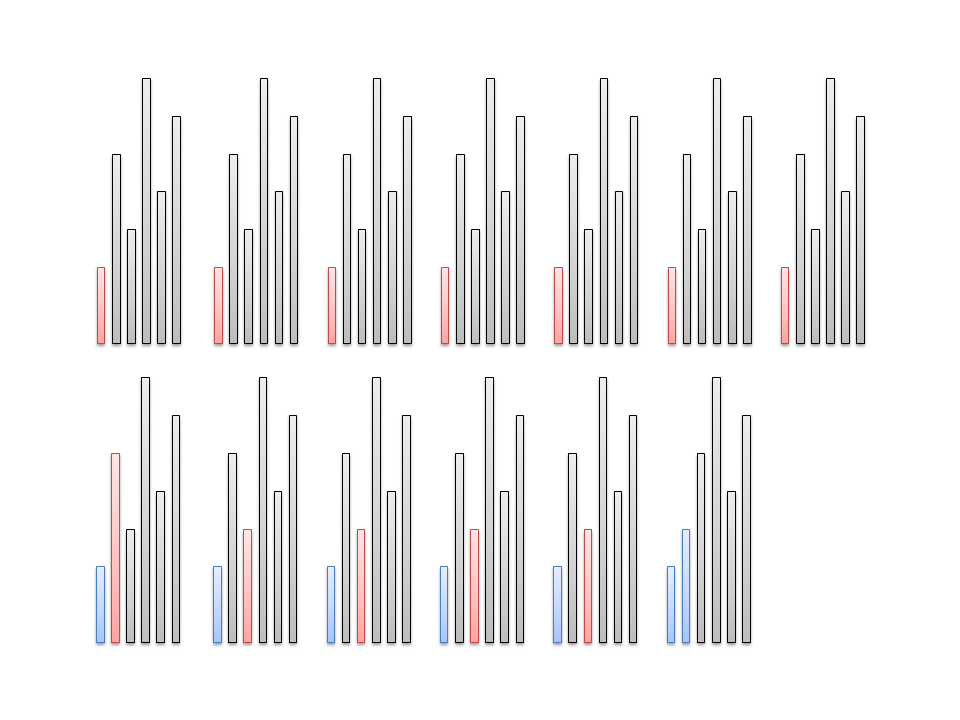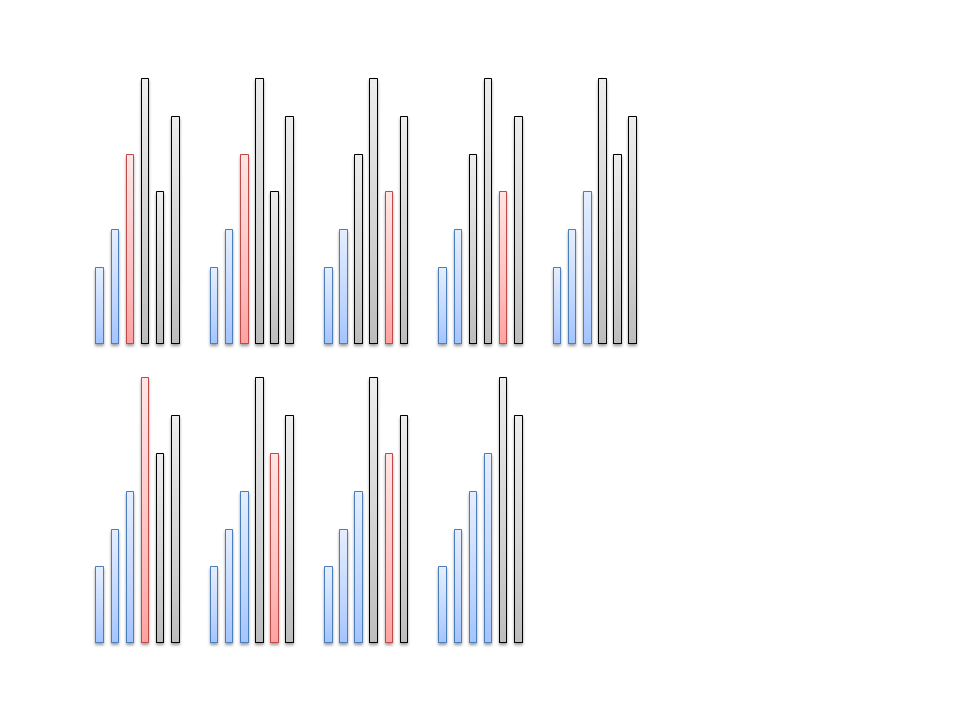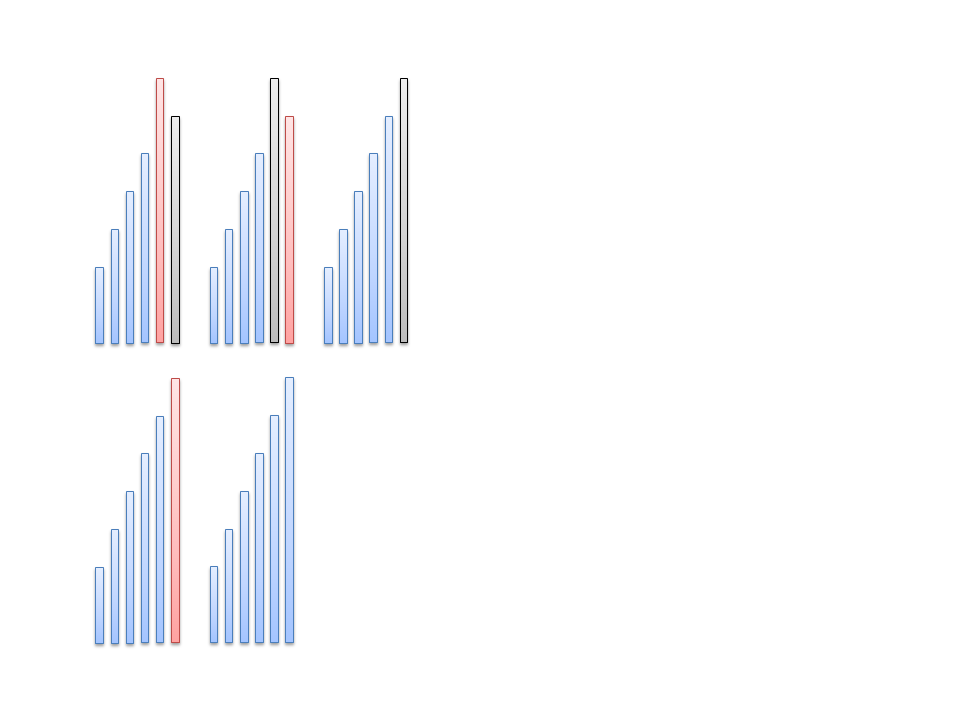# 选择排序

``````for i in 0..a.len(){
let mut min=i;
for j in i+1..a.len(){
if a[j]<a[min]{
min=j;
}
}
let temp=a[i];
a[i]=a[min];
a[min]=temp;
}
for i in &a{
print!("{} ",i);
}``````